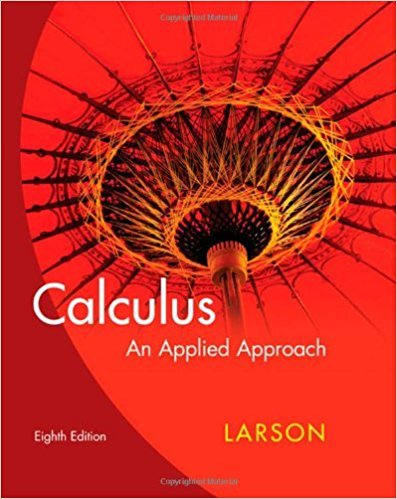×
×

# Solutions for Chapter 1.5: Limits## Full solutions for Calculus: An Applied Approach | 8th Edition

ISBN: 9780618958252Solutions for Chapter 1.5: Limits

Solutions for Chapter 1.5
4 5 0 339 Reviews
18
5
##### ISBN: 9780618958252

Since 73 problems in chapter 1.5: Limits have been answered, more than 22547 students have viewed full step-by-step solutions from this chapter. This textbook survival guide was created for the textbook: Calculus: An Applied Approach , edition: 8. Chapter 1.5: Limits includes 73 full step-by-step solutions. This expansive textbook survival guide covers the following chapters and their solutions. Calculus: An Applied Approach was written by and is associated to the ISBN: 9780618958252.

Key Calculus Terms and definitions covered in this textbook
• Complex conjugates

Complex numbers a + bi and a - bi

• Conversion factor

A ratio equal to 1, used for unit conversion

• Decreasing on an interval

A function f is decreasing on an interval I if, for any two points in I, a positive change in x results in a negative change in ƒ(x)

• equation of a quadratic function

ƒ(x) = ax 2 + bx + c(a ? 0)

• Finite series

Sum of a finite number of terms.

• Graph of parametric equations

The set of all points in the coordinate plane corresponding to the ordered pairs determined by the parametric equations.

• Left-hand limit of f at x a

The limit of ƒ as x approaches a from the left.

• Lemniscate

A graph of a polar equation of the form r2 = a2 sin 2u or r 2 = a2 cos 2u.

• Negative association

A relationship between two variables in which higher values of one variable are generally associated with lower values of the other variable.

• Parameter interval

See Parametric equations.

• Polar axis

See Polar coordinate system.

Any one of the four parts into which a plane is divided by the perpendicular coordinate axes.

• Resistant measure

A statistical measure that does not change much in response to outliers.

• Response variable

A variable that is affected by an explanatory variable.

• Subtraction

a - b = a + (-b)

• Sum of complex numbers

(a + bi) + (c + di) = (a + c) + (b + d)i

• Time plot

A line graph in which time is measured on the horizontal axis.

• Upper bound test for real zeros

A test for finding an upper bound for the real zeros of a polynomial.

• Vector equation for a line in space

The line through P0(x 0, y0, z0) in the direction of the nonzero vector V = <a, b, c> has vector equation r = r0 + tv , where r = <x,y,z>.

• Ymax

The y-value of the top of the viewing window.

×